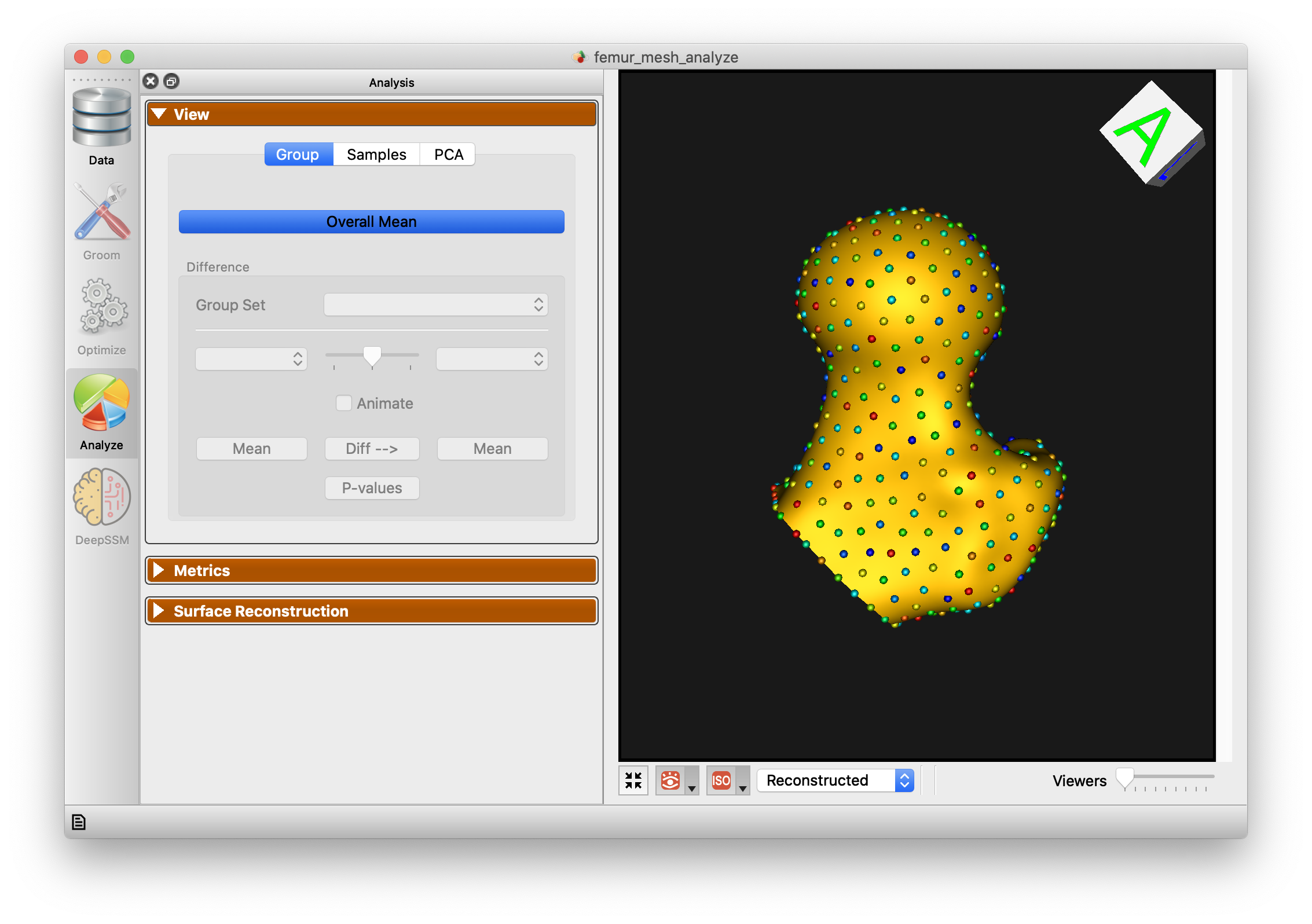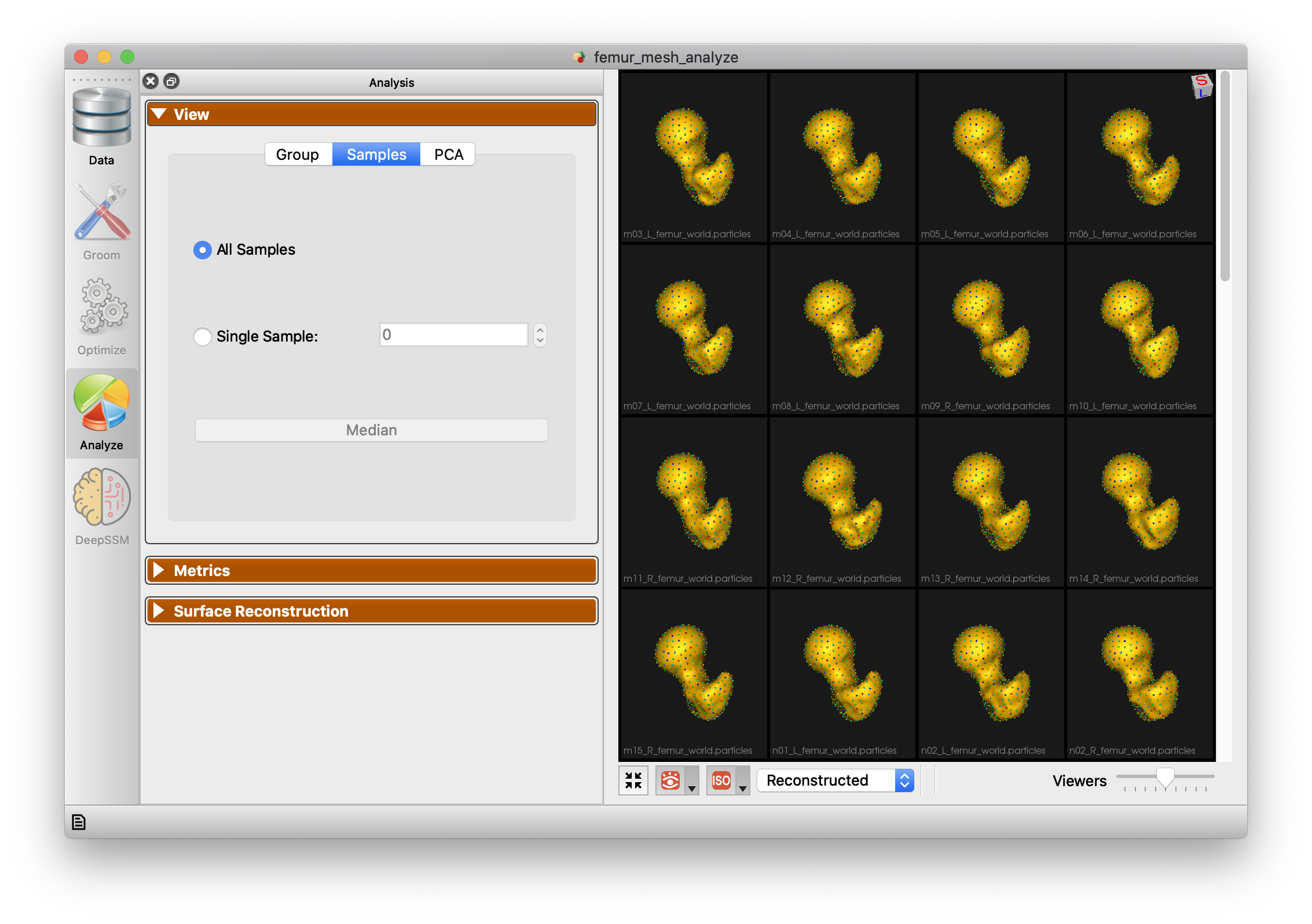# Femur-Mesh: Shape Model directly from Mesh

## What and Where is the Use Case?

This use case demonstrates using ShapeWorks tools to perform the following.

• Build a shape model where shapes are given as triangular surface meshes
• Groom a dataset from meshes, and obtain a SSM without converting the meshes to a different representation. This requires significantly lower RAM.

The use case is located at: Examples/Python/femur_mesh.py## Running the Use Case

To run the use case, run RunUseCase.py (in Examples/Python/).

$cd /path/to/shapeworks/Examples/Python$ python RunUseCase.py femur_mesh


This calls femur_mesh.py (in Examples/Python/) to perform the following.

• Optimizes particle distribution (i.e., the shape/correspondence model) by calling optimization functions in OptimizeUtils.py (in Examples/Python/). See Optimizing Shape Model for details about algorithmic parameters for optimizing the shape model.
• Launches ShapeWorks Studio to visualize the use case results (i.e., the optimized shape model and the groomed data) by calling functions in AnalyzeUtils.py (in Examples/Python/).

## Grooming Data

In this use case, we download pre-groomed data. The use case will be updated soon to demonstrate the full mesh grooming process.

## Optimizing Shape Model

Below are the default optimization parameters when running this use case. For a description of the optimize tool and its algorithmic parameters, see: How to Optimize Your Shape Model.

\$ python RunUseCase.py femur_mesh

{
"number_of_particles": 1024,
"use_shape_statistics_after": 0,
"use_normals": 0,
"normal_weight": 0.0,
"checkpointing_interval" : 10000,
"keep_checkpoints" : 0,
"iterations_per_split" : 4000,
"optimization_iterations" : 500,
"starting_regularization" : 10,
"ending_regularization" : 1,
"recompute_regularization_interval" : 1,
"domains_per_shape" : 1,
"domain_type" : 'mesh',
"relative_weighting" : 10,
"initial_relative_weighting" : 1,
"procrustes_interval" : 1,
"procrustes_scaling" : 1,
"save_init_splits" : 0,
"verbosity" : 2,
}


## Analyzing Shape Model

ShapeWorks Studio visualizes/analyzes the optimized particle-based shape model by visualizing the mean shape, individual shape samples, and the shape modes of variations. For more information, see: How to Analyze Your Shape Model?.

Here is the mean shape of the optimized shape mode using single-scale optimization.Here are femur samples with their optimized correspondences.Here is a video showing the shape modes of variation (computed using principal component analysis - PCA) of the femur dataset using single-scale optimization.